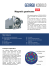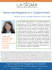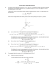# PHYS1250 Fundamental Physics Assignment Three Due Date: 5

## Transcription

PHYS1250 Fundamental Physics Assignment Three Due Date: 5
```PHYS1250 Fundamental Physics
Assignment Three
Due Date: 5:00p.m., November 28, 2014
1. An electron with kinetic energy 1.20 keV circles in a plane perpendicular to a uniform magnetic field. The
radius of orbit is 25.0 cm and the mass of electron is 9.11 × 10−31 kg. Find
(a) The speed of the electron,
(b) the magnetic field,
(c) the frequency of circling, and
(d) the period of the motion.
2. Three point charges are placed in a line as shown in the figure.
If r >> d, show that the potential V (r) at point P is given by
q
2d
V (r) =
1+
.
4πr
r
You may consider the binomial approximation (1 + x)n ≈ 1 + nx, where |x| << 1.
3. Two long wires are oriented so that they are perpendicular to each other. At their closest, they are 20.0 cm
apart. What is the magnitude of the magnetic field at a point midway between them if the top one carries a
current of IT = 20.0 A and the bottom one carries IB = 5.0 A?
4. The area of a 120-turn coil oriented with its plane perpendicular to a 0.20-T magnetic field is 0.050 m2 . Find
the average induced emf in this coil if the magnetic field reverses its direction in 0.34 s.
5. Light of wavelength 480 nm in air falls on two slits 6.00 × 10−2 mm apart. The slits are immersed in water and
they are located at a distance 40.0 cm away from a viewing screen which is also under water. The refractive
index of water is 1.33. How far apart are the fringes on the screen?
6. A thin film of alcohol (n = 1.36) lies on a flat glass plate (n = 1.51). When monochromatic light, whose
wavelength can be changed, is incident normally, the reflected light is a minimum for λ = 512 nm and a
maximum for λ = 640 nm. What is the thickness of the film?
7. Light is incident on a diffraction grating with 8600 lines/cm, and the pattern is viewed on a screen 2.5 m from
the grating. The incident light beam consists of two wavelengths, λ1 = 4.6 × 10−7 m and λ2 = 6.8 × 10−7 m.
Calculate the linear distance between the first-order bright fringes of these two wavelengths on the screen.
8. In a single-slit diffraction, the second minimum in the diffraction pattern occurs at 0.70◦ . If the slit width is
0.10 mm, what is the wavelength (in mm) of the light?
END OF PAPER
```

### Key HW5_2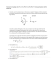### Medizinische Austellung A4 Wien 2008.indd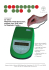### PHYSQ 126, Quiz 6 (19 mars 2015) Interaction of a Current Loop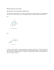### Problem Set 08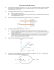### PHYA2 INT DIFF_Q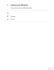### Ph 632 Problem Set #4 - Department of Physics | Oregon State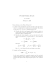### Electromagnetic AP Problems Worksheet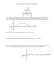### Key Homework 5.4. 1. a. A direct current I flows in a straight wire of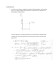### Phys 208 - Recitation E-Fields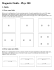### ME-709 Magnetic Recumbent Exercise Bike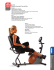### Frontier of Spintronics and Magnetic Sensing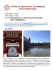### CMB Example SECTION A-type questions. Band theory of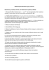### Abstract - MAGNETISM.eu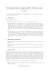### NATIONAL UNIVERSITY OF SINGAPORE DEPARTMENT OF PHYSICS ADVANCED PLACEMENT TEST (SAMPLE)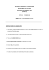### Micromag Datasheet - Eclipse Magnetics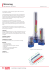### EXPERIMENT 1: SPECIFIC CHARGE OF THE ELECTRON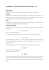### Experimental verifications Davisson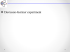### Are You Stuck on 3D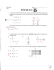### a PDF of this post with nicer formatting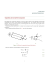### GEORGIIKOBOLD